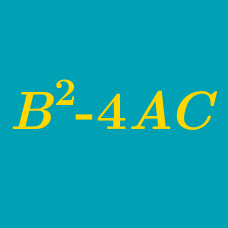Algebra

# Quadratic Discriminant - Determining Types of Roots

Which of the following equations has two distinct real roots?

$\begin{array}{c}&(A)~x^2-x+1=0 &&(B)~16x^2+8x+1=0\\ (C)~\frac{2}{5}x^2+\frac{3}{10}x+\frac{1}{5}=0 &&(D)~x^2+5x+4=0 \end{array}$

If $a < 3,$ which of the following is true about the quadratic equation: $x^2+2ax+a^2-3a+11=0 ?$

If the quadratic equation $x^2+7x-k=0$ has real roots, and the quadratic equation $x^2-2x-k=0$ has non-real complex roots, what is the range of $k?$

How many distinct real roots does the following equation have: $(x^2+x+4)(x^2+6x+8)=0?$

Which of the following is true about the quadratic equation: $41x^2-20x+4=5x^2+4x?$

×